# Mathematical Slight of Hand: Special Relativity and the Light-like Separated Events Equation Part 2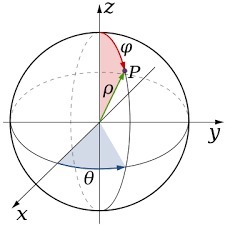There’s a mathematical riddle that’s been floating around for a while now. It tells the story of three men staying at a hotel room, each paying 10 dollars to stay there. The manager then realizes that he overcharged the men by 5 dollars, and tells his busboy to give the men the surplus money back to them. Instead, the Busboy pockets 2 dollars and gives 1 dollar back to each one.

Now that the three men have received a dollar back, that means that they’ve each paid 9 dollars. But if you do a multiplication of that number and add in the 2 dollars that the Busboy kept, you end up losing a dollar.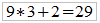So where’s the extra dollar?

The answer is that the above formulation is flawed in its construction. It changes the action from what the room cost to what the men paid and adds in the two dollars of cost to the different argument, which is faulty logic. Instead of adding that number to the total, it should be subtracted instead. Then the original left over total five dollars should be added back into the total and you get the expected 30 dollars.

Recall, then, that in the last article I wrote, I concluded that the ‘light-like separated events’ equation is formulated on faulty logic, stating that c has both a translation element and a time element which puts the equation in trouble from the start. We’ll start with a form of the equation that is more consistent with the Pythagorean Theorem: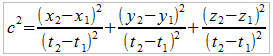And then I argued that c has both a translation and a time element in it, so you can rightly express the above equation like this.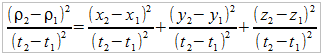This is because the left-hand term is simply the result of a simple 3-D Pythagorean Formulation of the Velocity components of a light-ray in a vacuum. The left-hand side is known as speed. It is a scalar value, meaning that there is no direction associated with it. As mentioned before, the changes in x, y, and z values are vector components in Cartesian-coordinates.

The mathematicians try to get around the left-hand side by an abstraction. They state that c, the speed of light in a vacuum, is constant no matter where it is in space and no matter what object a photon of light is emitted from. Therefore, you can replace the translation and time components with a simple number, and thus, once again:It is from this abstraction that the mathematician gains the rationale to treat time as the fourth dimension and they come up with the concept known as ‘space-time’.

Here’s the problem.

I told you that the right side of this equation is composed of x, y, and z vector components in a Cartesian-coordinate system. But the right side of the equation is not a vector. It is a dot-product of the vector, which produces a scalar. You can’t add vectors and end up with a scalar on the right-hand side. That’s why the components on the right-hand side are squared. There are no dimensions on the right-hand.

The mathematician uses a slight of hand by only producing the radial component of a 3-D radial coordinate system. The variable, or c, in our case, is just a raw value that denotes the speed of the photon. It doesn’t say anything about the direction that photon is going. So the mathematician only partially does the conversion from Cartesian-Coordinates to Spherical-Coordinates, or 3-D Polar Coordinates. To complete this calculation, you must calculate the following angles: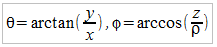Whereis already calculated from Pythagorean’s Theorem. The new Spherical Vector is then expressed as the scalar value plus the two additional angles needed specify the direction of the photon: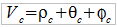This is a vector in 3-D space in spherical components, unlike the light-like separated events, which is a scalar equation.

Additionally, you can bring about the mathematicians abstraction of c to it’s logical completion by simply acknowledging that the right-hand side of the light-like separated events can be expressed as Cartesian-coordinate conversions of the polar radius.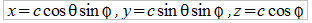So,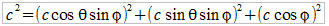Mathematics are very notorious for having an “anything goes” attitude for defining its own reality. It’s how it justifies the existence of time as a fourth dimension in our universe. The way that the light-like separated events equation was derived should be treated carefully and with much skepticism, especially when it claims to be describing real events in the Universe.